## 散射矩阵的计算

$\langle \bm{p}_1\cdots \bm{p}_n|S|\bm{p}_{\mathcal{A}}\bm{p}_{\mathcal{B}}\rangle = \langle \bm{p}_1\cdots \bm{p}_n|T\{\exp[-i\int_{-\infty}^{\infty}dtH_I(t)]\}|\bm{p}_{\mathcal{A}}\bm{p}_{\mathcal{B}}\rangle \tag{1}$

$\langle \bm{p}_1\bm{p}_2|T\{\exp[-i\int_{-\infty}^{\infty}dtH_I(t)]\}|\bm{p}_{\mathcal{A}}\bm{p}_{\mathcal{B}}\rangle \tag{2}$

\begin{aligned} \langle \bm{p}_1\bm{p}_2|\bm{p}_{\mathcal{A}}\bm{p}_{\mathcal{B}}\rangle &= \sqrt{2E_12E_22E_{\mathcal{A}}2E_{\mathcal{B}}}\langle 0| \hat{a}_{\bm{p}_1}\hat{a}_{\bm{p}_2}\hat{a}^\dagger_{\bm{p}_{\mathcal{A}}}\hat{a}^\dagger_{\bm{p}_{\mathcal{B}}}|0\rangle \\ &= 2E_{\mathcal{A}}2E_{\mathcal{B}}(\delta^{(3)}(\bm{p}_{\mathcal{A}}-\bm{p}_1)\delta^{(3)}(\bm{p}_{\mathcal{B}}-\bm{p}_2) + \delta^{(3)}(\bm{p}_{\mathcal{A}}-\bm{p}_2)\delta^{(3)}(\bm{p}_{\mathcal{B}}-\bm{p}_1)) \end{aligned}

$S = 1 + iT \tag{3}$

$\langle \bm{p}_1\bm{p}_2|T\{-i\int dtH_I(t)\} |\bm{p}_{\mathcal{A}}\bm{p}_{\mathcal{B}}\rangle \tag{4}$

\begin{aligned} &\langle \bm{p}_1\bm{p}_2|T\{-i\int d^4z \phi^4(z)\} |\bm{p}_{\mathcal{A}}\bm{p}_{\mathcal{B}}\rangle\\ =& \langle \bm{p}_1\bm{p}_2|N\{-i\int d^4z \phi^4(z) + contractions\} |\bm{p}_{\mathcal{A}}\bm{p}_{\mathcal{B}}\rangle\\ \end{aligned}\tag{5}

\begin{aligned} \langle \bm{p}_1\bm{p}_2|\bm{p}_{\mathcal{A}}\bm{p}_{\mathcal{B}}\rangle = \langle \bm{p}^{\bullet}_1\bm{p}^{\star}_2|\bm{p}^{\bullet}_{\mathcal{A}}\bm{p}^{\star}_{\mathcal{B}}\rangle + \langle \bm{p}^{\bullet}_1\bm{p}^{\star}_2|\bm{p}^{\star}_{\mathcal{A}}\bm{p}^{\bullet}_{\mathcal{B}}\rangle \end{aligned}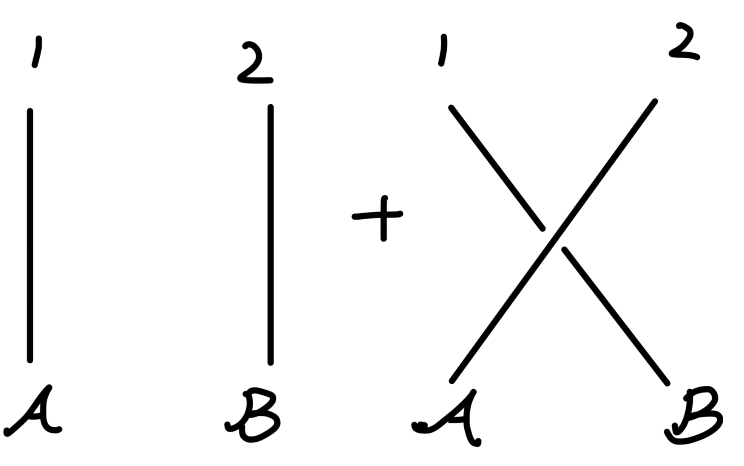Fig1：散射矩阵零阶项

\begin{aligned} \phi^{+}_I(x)|\bm{p}\rangle &= \int \frac{d^3k}{(2\pi)^3} \frac{1}{\sqrt{2E_{\bm{k}}}} \hat{a}_{\bm{k}}e^{-ik\cdot x} \sqrt{2E_{\bm{p}}} \hat{a}_{\bm{p}}^\dagger |0\rangle\\ &= \int d^3k \frac{1}{\sqrt{2E_{\bm{k}}}} e^{-ik\cdot x} \sqrt{2E_{\bm{p}}} \delta(\bm{k}-\bm{p}) |0\rangle\\ &= e^{-ip\cdot x} |0\rangle\\ \end{aligned}\tag{6}

$\langle \bm{p}|\phi^{-}_I(x) = \langle 0|e^{ip\cdot x} \tag{7}$

\begin{aligned} \phi^{\bullet}_I(x)|\bm{p}^{\bullet}\rangle &= e^{-ip\cdot x} |0\rangle\\ \langle \bm{p}^{\bullet}|\phi^{\bullet}_I(x) &= \langle 0|e^{ip\cdot x}\\ \end{aligned}\tag{8}

$\phi\phi\phi\phi;\quad \phi^{\bullet}\phi^{\bullet}\phi\phi;\quad \phi^{\bullet}\phi^{\bullet}\phi^{\bullet\bullet}\phi^{\bullet\bullet};$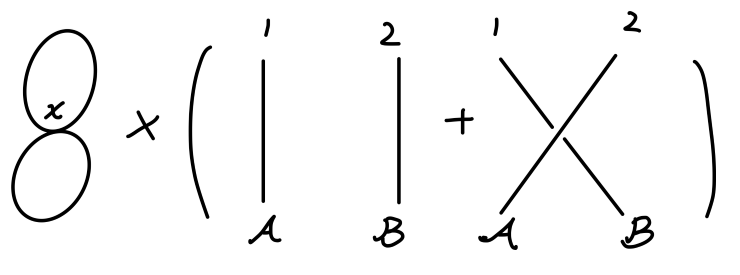Fig2：散射矩阵一阶项：类型3

• $\phi_I^{\bullet}(x)|\bm{p}^{\bullet}\rangle$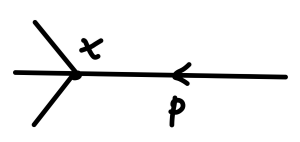Fig3：场量与末态缩并

• $\langle \bm{p}^\bullet|\phi_I^{\bullet}(x)$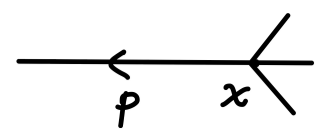Fig4：场量与初态缩并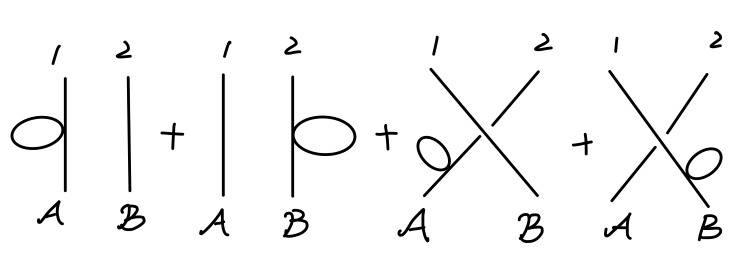Fig5：散射矩阵一阶项：类型2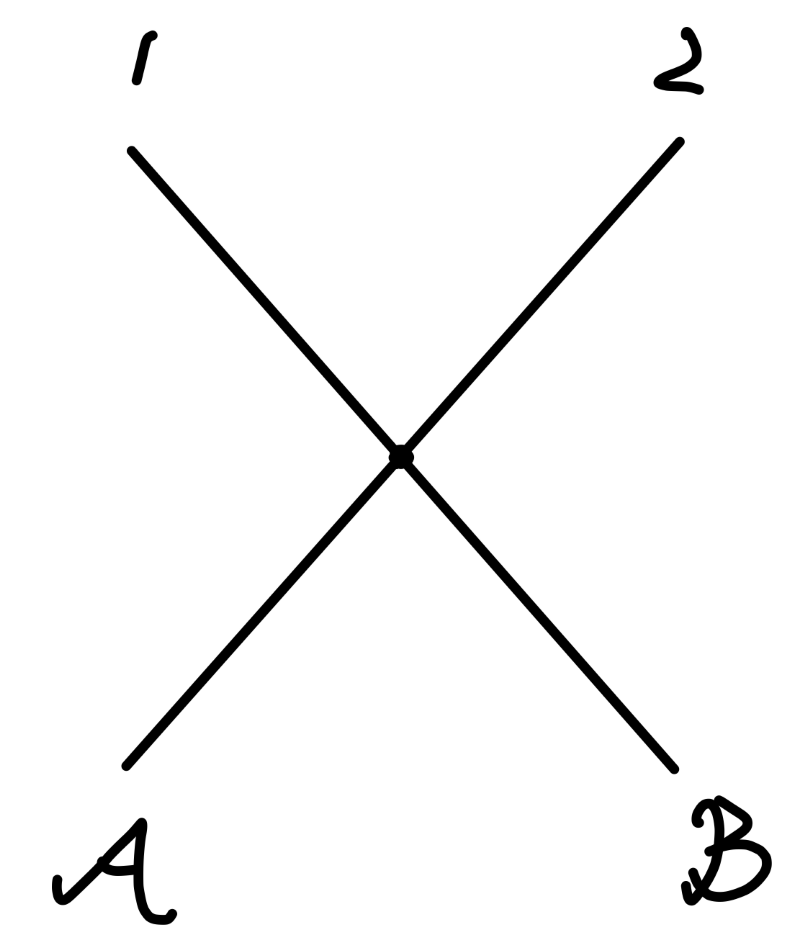Fig6：散射矩阵一阶项：类型1

\begin{aligned} &(4!)\cdot(-i\frac{\lambda}{4!})\int d^4x e^{-i(p_{\mathcal{A}}+p_{\mathcal{B}}-p_1-p_2)\cdot x}\\ =& -i\lambda (2\pi)^4 \delta^{(4)}(p_{\mathcal{A}}+p_{\mathcal{B}}-p_1-p_2) \end{aligned}

$\langle \bm{p}_1 \bm{p}_2|iT|\bm{p}_{\mathcal{A}}\bm{p}_{\mathcal{B}}\rangle$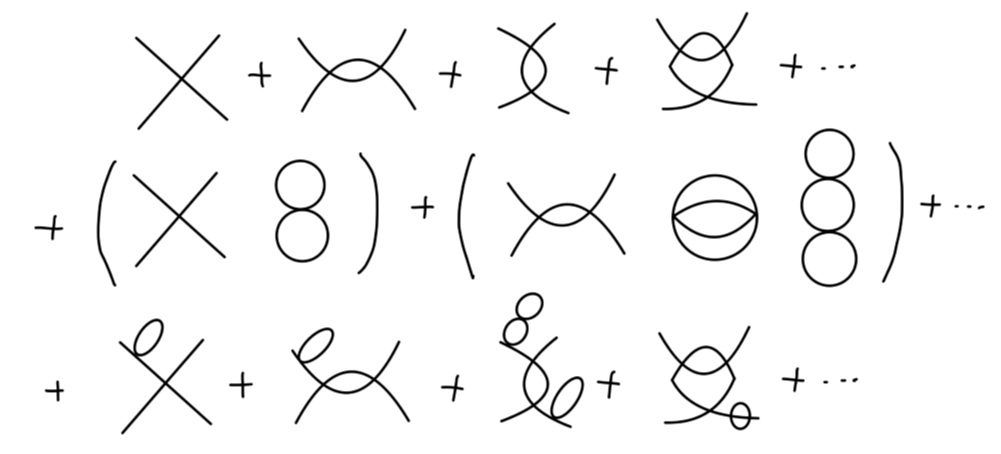Fig：散射矩阵

$\exp(\sum_{i} V_i)$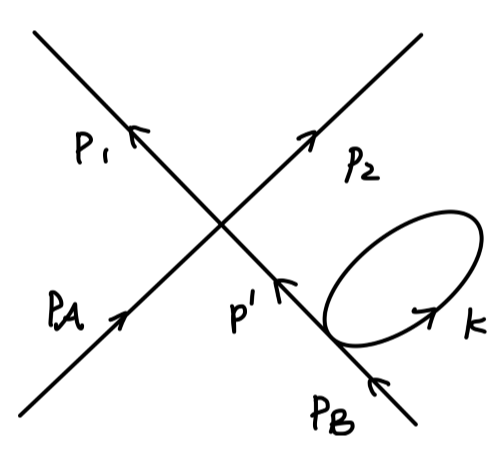\begin{aligned} &\frac{1}{2}\int \frac{d^4p'}{(2\pi)^4} \frac{i}{p'^2 - m^2} \int \frac{i}{k^2-m^2}\\ &\times(-i\lambda)(2\pi)^4\delta^{(4)}(p_\mathcal{A} + p' - p_1 -p_2)\\ &\times (-i\lambda)(2\pi)^4\delta^{(4)}(p_\mathcal{B} - p')\\ \end{aligned}

$\frac{i}{p'^2-m^2}|_{p' = p_{\mathcal{B}}} = \frac{i}{p_{\mathcal{B}}^2 - m^2} = \frac{i}{0}$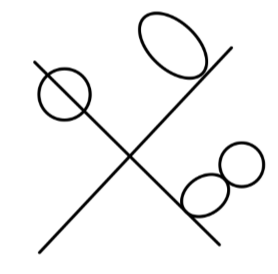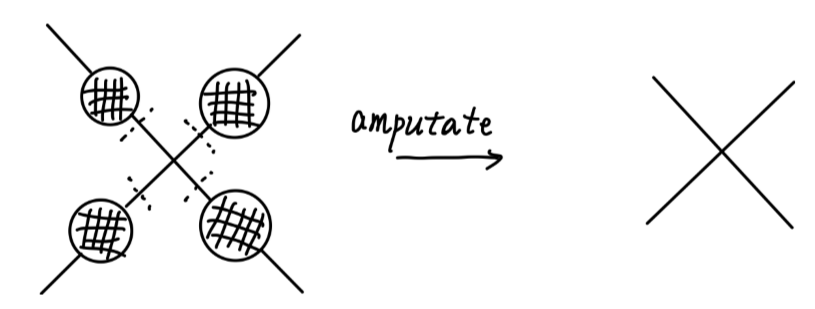\langle \bm{p}_1 \bm{p}_2|iT|\bm{p}_{\mathcal{A}}\bm{p}_{\mathcal{B}}\rangle = \sum \left\{ \begin{aligned} &fully\ connected\ \\ &utated\ diagram\ \\ \end{aligned}\right\} \tag{9}

### 费曼规则

• 传播子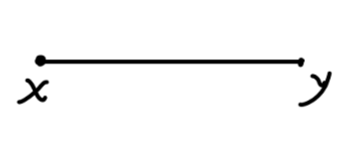$D_F(x-y)$

• 顶点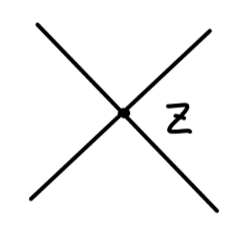$(-i\lambda) \int d^4z$

• 外部线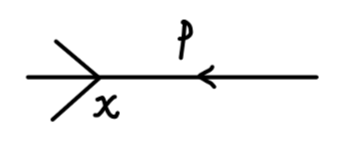$e^{-ip\cdot x}$

• 除以对称因子 $S$

• 传播子$\frac{i}{p^2 - m^2 + i\epsilon}$

• 顶点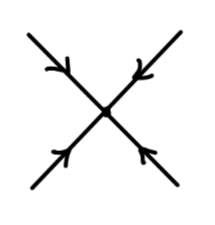$-i\lambda$

• 外部线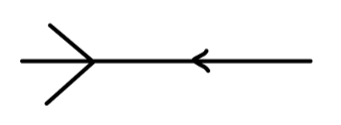$1$

• 顶点保证四动量守恒。

• 对未确定的动量进行积分：

$\int \frac{d^4p}{(2\pi)^4}$

• 除以对称因子 $S$